## Ratio and Proportion: Formulas, Tricks, Examples and Online Test

Ratio: Ratio is the spoken language of arithmetic. It is the language with which we relate quantities of the same kind.

15 people, for example, are more than 5 people. We can express that by saying how much more, that is, 10 more, or by saying how many times more: Three times more. When we say that 15 people are three times more than 5 people, that is called their ratio.

• The ratio of two terms ‘x’ and ‘y’ is denoted by x : y.
• In general, the ratio of a number x to a number y is defined as the quotient of the numbers x and y.
• The numerator of the ratio is called the antecedent (x) and the denominator is called the consequent (y) of the ratio.

### Properties of Ratio

a : b = m a : m b, where m is a constant

a : b : c = A : B : C is equivalent to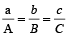,

This is an important property and has to be used in ratio of three things.

If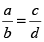then,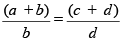This property is called Componendo

For example: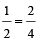So,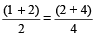=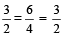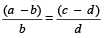This property is called Dividendo

For example: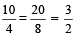So,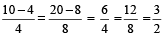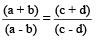This property is called Componendo and Dividendo

For example: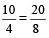So,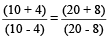=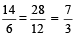If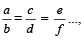then,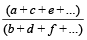= each of the individual ratio

For example: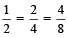Therefore,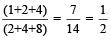If A > B then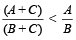Where A, B and C are natural numbers

For example: 3 > 2, then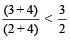[ as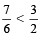]

If A < B then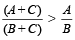Where A, B and C are natural numbers.

For example: 2 < 3, then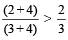[ as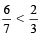]

### To divide a given quantity into a given ratio

Suppose any given quantity a, is to be divided in the ratio m : n.

Let one part of the given quantity be x then the other part will be a – x.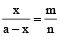or nx = ma – mx

or (m + n) x = ma

∴ one part is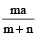and the other part will be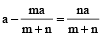Example 1: Divide 70 in the ratio 3 : 7

Solution: Let one part be x, then the other part = 70 – x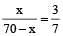or 7x = 210 – 3x

or x = 21 and 70 – x = 49

Hence the two required parts of 70 are 21 and 49.

In any 2-dimensional figures, if the corresponding sides are in the ratio x : y, then their areas are in the ratio x² : y².

Example 2: The ratio of the radius of two circles is 2 : 5. Find the ratio of their areas.

Solution: Ratio of their areas = 2² : 5² = 4 : 25

In any two 3-dimensional figures, if the corresponding sides are in the ratio x : y, then their volumes are in the ratio x³ : y³. If the ratio between two numbers is a : b and

if each number is increased by x, the ratio becomes c : d. Then

Sum of the two numbers

=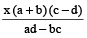Difference of the two numbers

=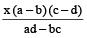Two numbers are given as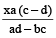and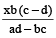Example 3: The ratio between two numbers is 3 : 4. If each number be increased by 2, the ratio becomes 7 : 9. Find the numbers.

Solution:

Numbers are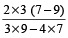and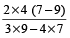or 12 and 16.

If the sum of two numbers is A and their difference is a, then the ratio of numbers is given by A + a : A – a.

Example 4: The sum of two numbers is 60 and their difference is 6. What is the ratio of the two numbers?

Solution: The required ratio of the numbers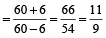or 11 : 9

### Proportion

Proportion is an expression in which two ratios are equal.

For example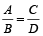,

⇒ A : B : : C : D

Here,  A D = B C

Example 5: If 12 : 18 :: x : 24, then find the value of x.

Solution: 12 : 18 : : x : 24

⇒ 12 × 24 = 18 × x

⇒ x =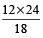= 16

### Type of Proportion

Continued Proportion: If three numbers a, b and c are in continued proportion,then:

We can say that a, b and c are in proportion.

i.e.,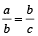⇒ b² = ac

⇒ b =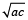Here we can say that a is called first proportion, c is called third proportion and b is called mean proportion.

Direct Proportion: If X is directly proportional to Y, that means any increase or decrease in any of two quantities will have proportionate effect on the other quantity. If X increases then Y will also increase and vice-versa.

Inverse Proportion: If X is inversely proportional to Y, that means any increase or decrease in any of two quantities will have inverse proportionate effect on the other quantity. This means if X increases, then Y decreases and if X decreases then Y increases and vice-versa for Y.

If four quantities are in proportion, the product of the extremes is equal to the product of the means.

Let a, b, c, d be in proportion, then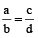If three quantities a, b and c are in continued proportion, then
a : b = b : c

∴ ac = b²

b is called mean proportional.

If three quantities are proportional, then first is to the third is the duplicate ratio of the first is to the second.

If a : b :: b : c then a : c = a² : b²

### To find the mean proportional

Example 6: Find the mean proportional between 3 and 75.

Solution: Let x be the required mean proportional. Then,

3 : x :: x : 75

∴ x =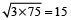### To find the values of an unknown when four numbers are in proportion

Example 7: What must be added to each of the four numbers 10, 18, 22, 38 so that they become in proportion?

Solution: Let the number to be added to each of the four numbers be x.

By the given condition, we get

(10 + x) : (18 + x) : : (22 + x) : (38 + x)

⇒ (10 + x) (38 + x) = (18 + x) (22 + x)

⇒ 380 + 48x + x² = 396 + 40x + x²

Cancelling x² from both sides, we get

380 + 48x = 396 + 40x

⇒ 48x – 40x = 396 – 380

⇒ 8x = 16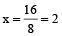Therefore, 2 should be added to each of the four given numbers.

### To find the fourth proportional

Example 8: Find the fourth proportional to p² – pq + q², p³ + q³, p – q

Solution: Let x be the fourth proportional

∴ (p²– pq + q²) : (p³ + q³) = (p – q) : x

⇒ (p² – pq + q²) × x = (p³ + q³) (p – q)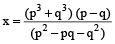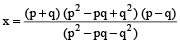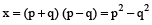∴ The required fourth proportional is p² – q²

### To find the third proportional

Example 9: Find third proportional to a² – b² and a + b.

Solution: Let x be the required third proportional

Then a² – b² : a + b = a + b : x

∴ (a² – b²) x = (a + b) (a + b)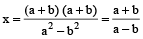### Using theorem on equal proportion

Example 10: If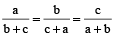, prove that each is equal to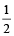or –1.

Solution: We have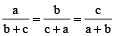Each ratio =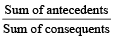[By theorem on equal ratios]

=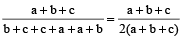=if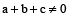If a + b + c = 0, then b + c = –a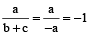Similarly,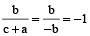,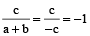Hence each ratio =if= –1 if a + b + c = 0